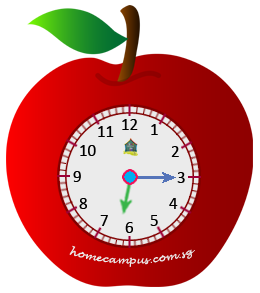Practice Unlimited Questions

#### How to read time on an analog clock?

Time is represented by Hours, Minutes and Seconds. For this grade we will only learn about Hours and Minutes.

If someone says that the time is 6:15, then 6 represents hours and 15 represents minutes. So the time is 15 minutes past 6. In other words, it is quarter past 6.

If the time is 6:45, then we can read it as 15 minutes to 6 or quarter to 7.

Look at the clock below. What is the time in the clock?The longer hand is the Minute hand.
The shorter hand is the Hour hand.

There are 5 minutes between two adjacent big markings on the clock.

The longer hand is at 3 that means, Minute hand is showing 3 × 5  =  15 minutes.

The hour hand is between 6 and 7.

So, the time is 6.15.

#### 2. Fill in the blank with 'past' or 'to'. Britney does the dishes at 7.15 pm. 7.15 is 15 minutes _______ 7.

7.15 is 15 minutes after 7 o'clock.

So, 7.15 is 15 minutes past 7.

#### 3. Fill in the blank with 'past' or 'to'. Carolina goes for a jog at 6.40 am. 6.40 is 20 minutes _______ 7.

6.40 is 20 minutes before 7 o'clock.

So, 6.40 is 20 minutes to 7.